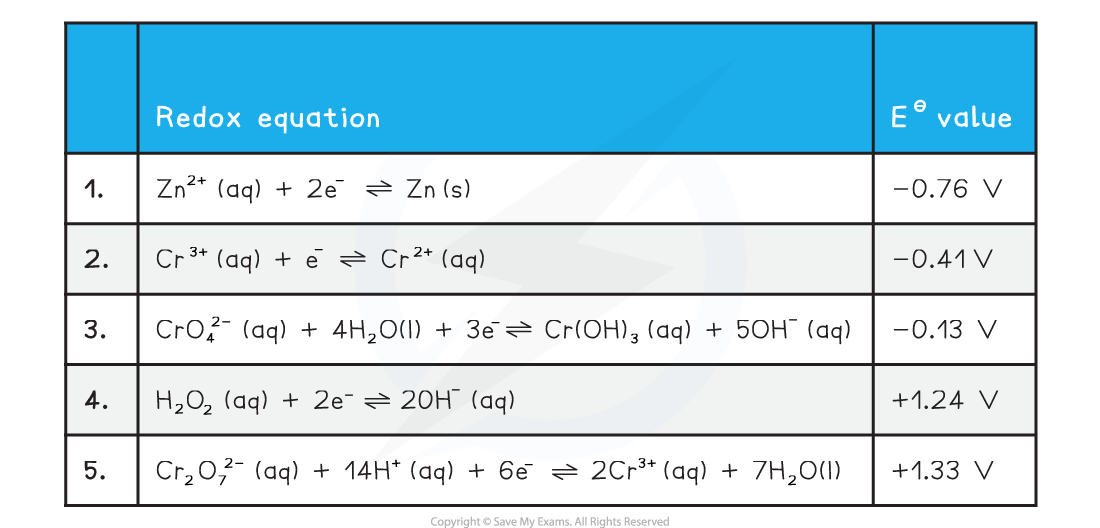# Edexcel A Level Chemistry:复习笔记6.3.2 Chromium

### Reduction & Oxidation of Chromium Species

• For chromium we need to consider the following standard electrode potential values
• We will use zinc and hydrogen peroxide as oxidising agents
• The half equations are arranged from high negative EΘ at the top to high positive EΘ at the bottom
• The best reducing agent is the top right species (Zn (s))
• The best oxidising agent is the bottom left species (Cr2O72-(aq))#### Oxidation from +3 to +6

• The two half equations we need to consider are 3 and 4
• Chromiums oxidation number changes from +6 to +3 in half equation 3
• The EΘ value for half equation 3 is more negative than the EΘ for half equation 4
• Cr(OH)3 (aq) is the best reducing agent
• H2O2 (aq) is the best oxidising agent
• We can obtain the overall equation by reversing half equation 3 and combining it with equation 4
• When adding half equations remember to multiply them so each has the same number of electrons

2Cr(OH)3 (aq) + 4OH- (aq) + 3H2O2 (aq) → 2CrO42- (aq) + 8H2O (l)

• This reaction is carried out in alkaline conditions due to the presence of OH- ions in the equation

#### Reduction from +6 to +3

• The two half equations we need to consider are 1 and 5
• Chromiums oxidation number changes from +6 to +3 in half equation 3
• The EΘ value for half equation 1 is more negative than the EΘ for half equation 5
• Zn is the best reducing agent
• Cr2O72- is the best oxidising agent
• We can obtain the overall equation by reversing half equation 1 and combining it with equation 5
• When adding half equations remember to multiply them so each has the same number of electrons

Cr2O72- (aq) + 14H+ (aq) + 3Zn (s) → 2Cr3+ (aq) + 7H2O (l) + 3Zn2+ (aq)

• This reaction is carried out under acidic conditions due to presence of H+ in the equation

#### Reduction from +3 to +2

• The Cr3+ ion can be further reduced by zinc
• The two half equations we need to consider are 1 and 2
• Chromiums oxidation number changes from +3 to +2 in half equation 3
• The EΘ value for half equation 1 is more negative than the EΘ for half equation 2
• Zn (s) is the best reducing agent
• Cr3+ (aq) is the best oxidising agent
• We can obtain the overall equation by reversing half equation 1 and combining it with equation 2
• When adding half equations remember to multiply them so each has the same number of electrons

2Cr3+ (aq) + Zn  (s) → 2Cr2+ (aq) + Zn2+ (aq)

• As this reaction is a further step from the previous reduction this reaction is also carried out under acidic conditions

### The Dichromate(VI) - Chromate(VI) Equilibrium

• The chromate CrO42- and dichromate Cr2O72- ions can be converted from one to the other by the following equilibrium reaction

2CrO42- (aq) + 2H+ (aq) ⇌ Cr2O72- (aq) + H2O (l)

• Chromate(VI) ions are stable in alkaline solution, but in acidic conditions the dichromate(VI) ion is more stable
• Addition of acid will push the equilibrium to the dichromate
• This results in a colour change from yellow to orange
• Addition of alkali will remove the H+ ions and push the equilibrium to the chromate
• This is not a redox reaction as both the chromate and dichromate ions have an oxidation number of +6
• This is an acid base reaction